In this topic we will explore temporal data in R in order to visualize the temporal aspect of porpoise distribution.

## 1. Dealing with time variables in R

As discussed previously, a variable is recognized as time in the POSIxct data type, for example by applying lubridate::parse_date_time().

A first step is to plot the value of interest against time.

``````ggplot(data = poddata_day, aes(x = Time, y = Dpm)) +
theme_bw() +
theme(axis.text = element_text(size = 16),
axis.title.x = element_blank()) +
geom_point() + geom_line()``````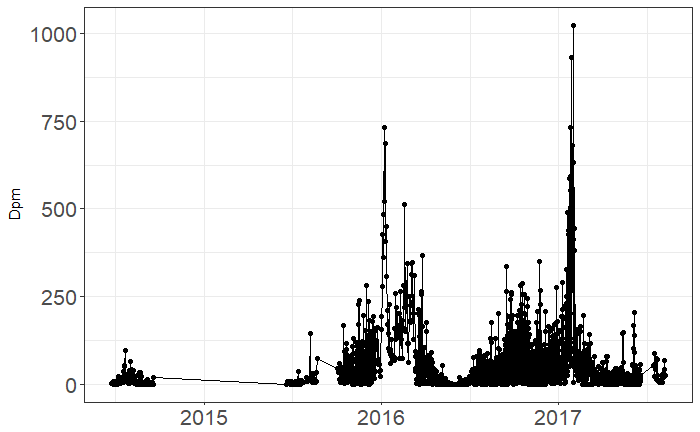More relevant is plotting the time series for each zone seperately. This can be done with facet_wrap() or by making a list of plots.

``````ggplot(data = poddata_day, aes(x = Time, y = Dpm)) +
theme_bw() +
theme(axis.text = element_text(size = 16),
axis.title.x = element_blank()) +
geom_point() + geom_line() + facet_wrap(~Zone, nrow = length(unique(poddata\$Station)))``````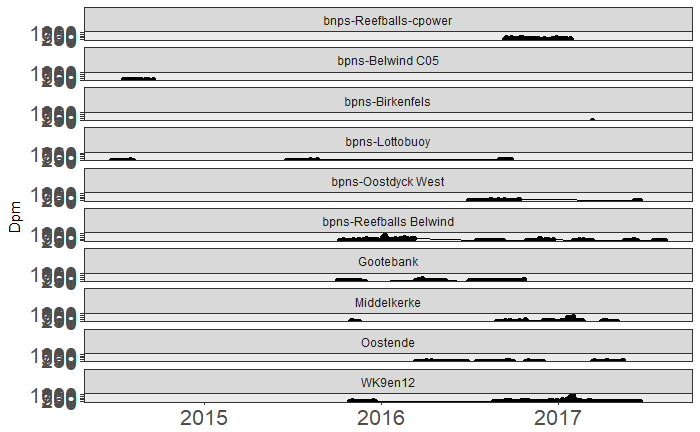``````lapply(unique(poddata_day\$Zone), function(x){
ggplot(data = poddata_day[poddata_day\$Zone == x,], aes(x = Time, y = Dpm)) +
theme_bw() +
theme(axis.text = element_text(size = 16),
axis.title.x = element_blank()) +
geom_point() + geom_line() + ggtitle(x)
})
``````
``````[]

[]

[]

[]

[]

[]

[]

[]

[]

[]``````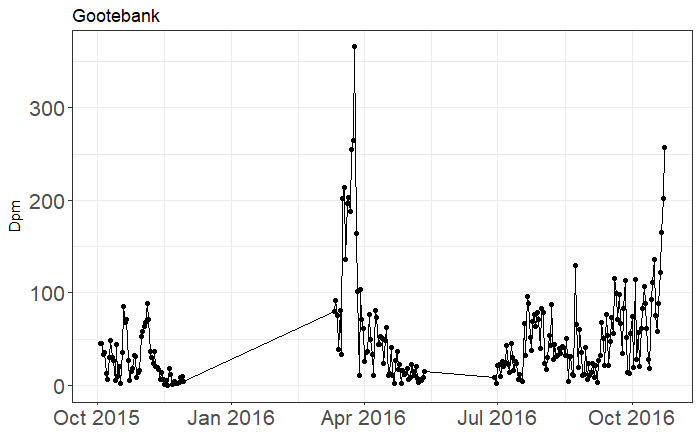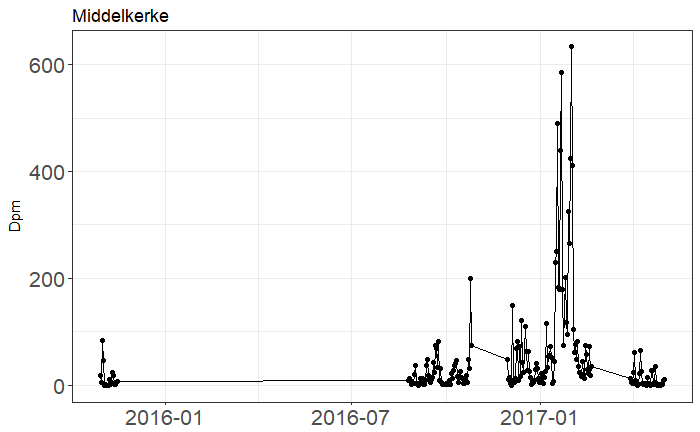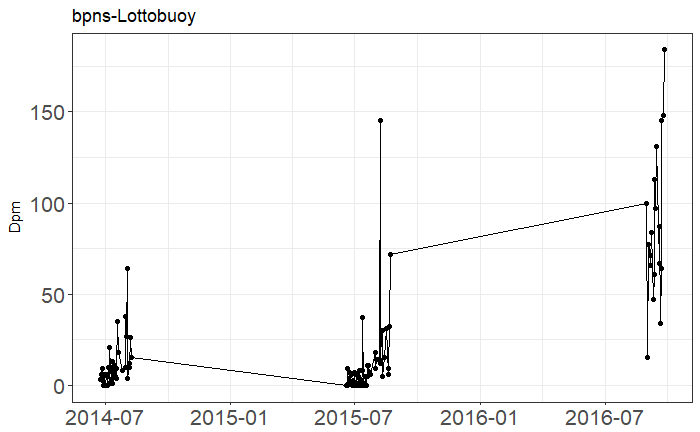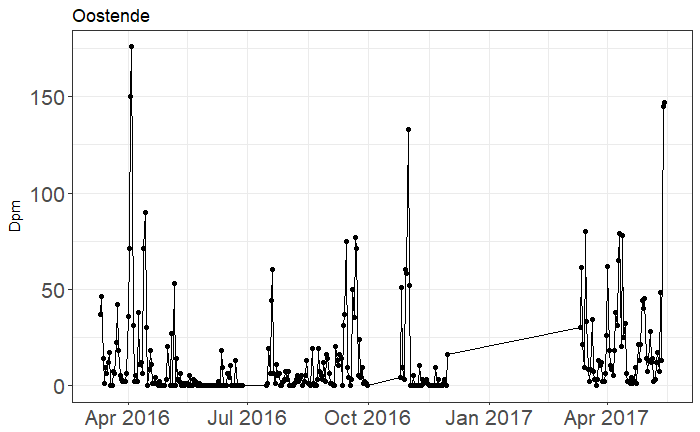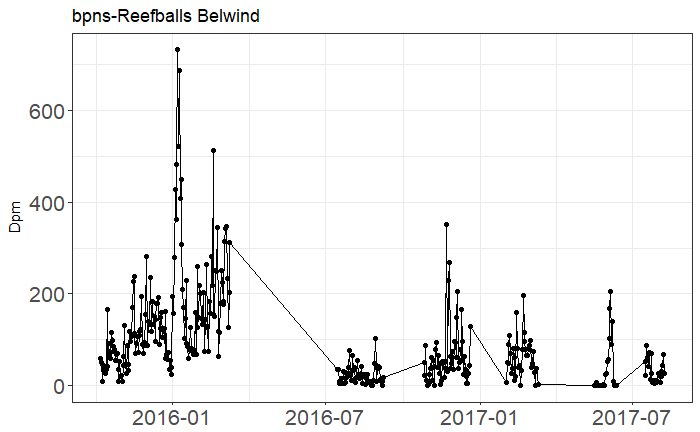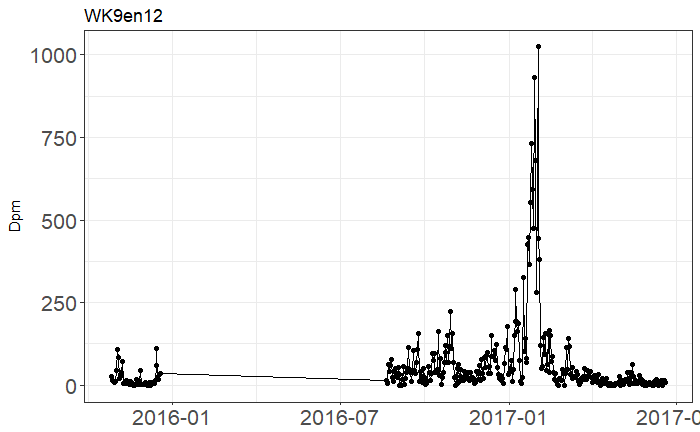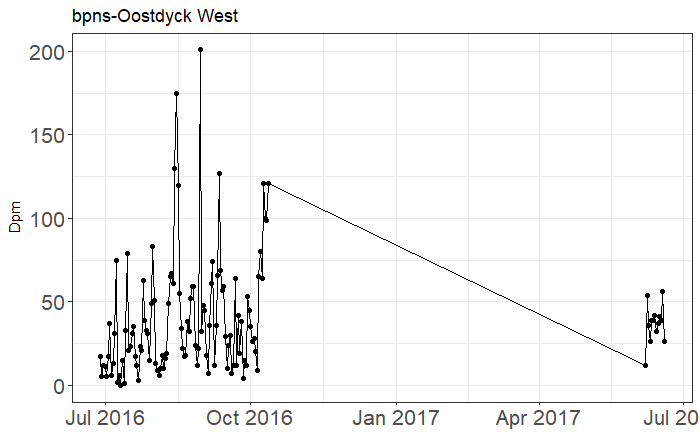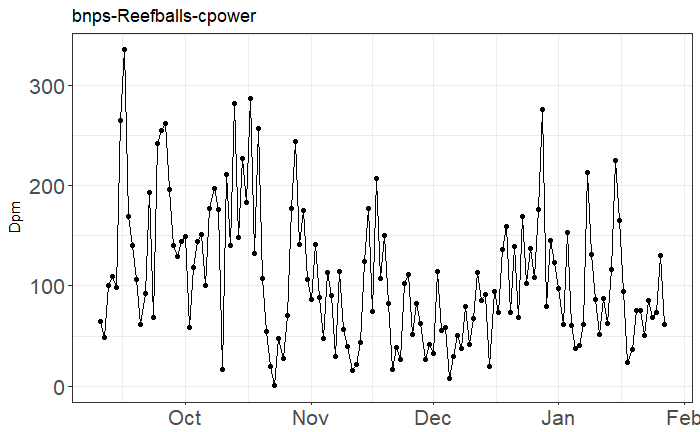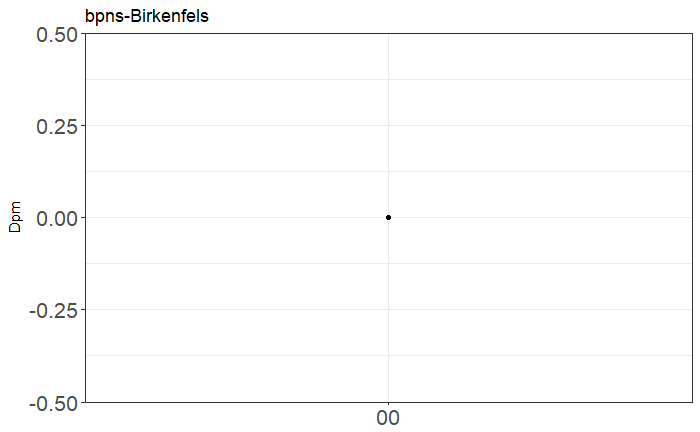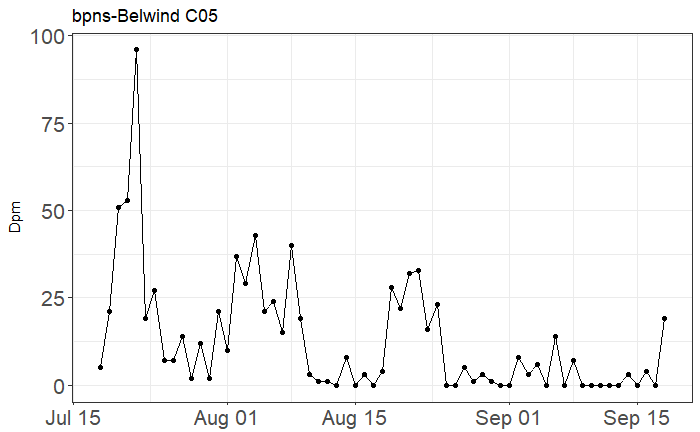Same can be done for different deployments.

``````lapply(unique(poddata_day\$Deployment_fk), function(x){
ggplot(data = poddata_day[poddata_day\$Deployment_fk == x,], aes(x = Time, y = Dpm)) +
theme_bw() +
theme(axis.text = element_text(size = 16),
axis.title.x = element_blank()) +
geom_point() + geom_line() + ggtitle(x)
})``````

## 2. Autocorrelation

R provides a very easy function to investigate the autocorrelation in your data: acf.

``````poddata_day <- arrange(poddata_day, Zone, Time)
acf(poddata_day\$Dpm)``````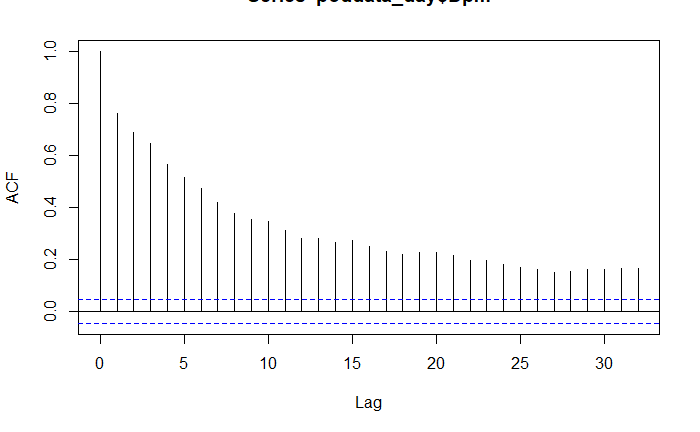Again, we are interested in the autocorrelation of each Zone seperately.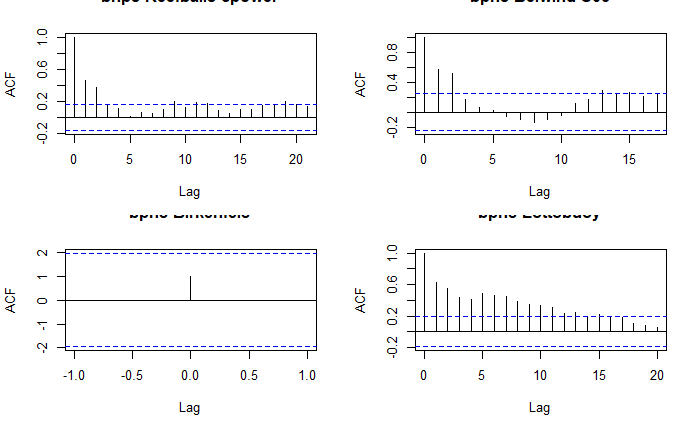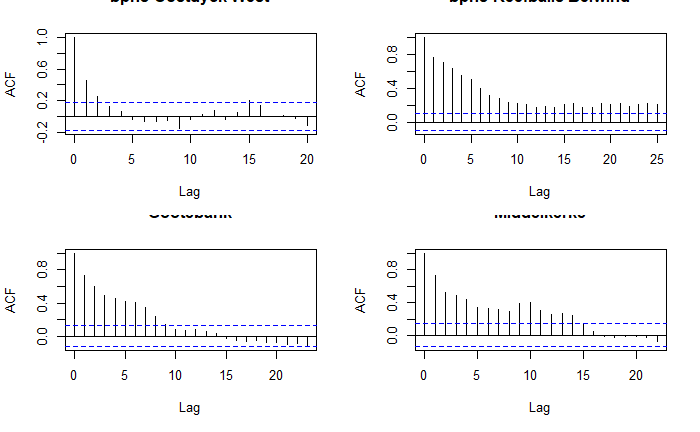``````par(mfrow=c(2,2)) # see four plots at a time
lapply(unique(poddata_day\$Zone), function(x) {
data <- acf(poddata_day[poddata_day\$Zone == x,]\$Dpm, plot = F)
plot(data, main = x)
})
``````
``````[]
NULL

[]
NULL

[]
NULL

[]
NULL

[]
NULL

[]
NULL

[]
NULL

[]
NULL

[]
NULL

[]
NULL``````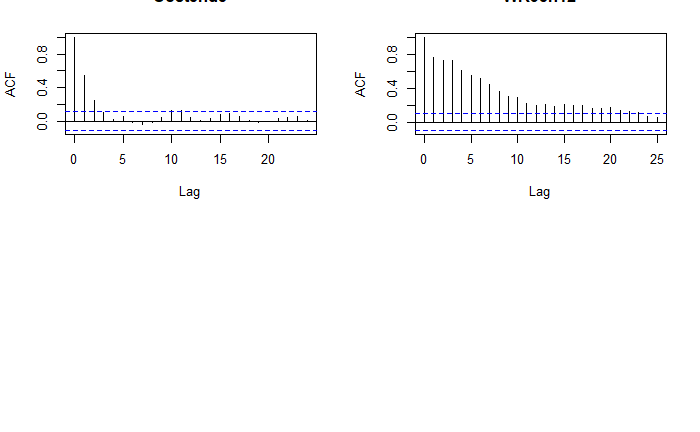We can also take these values and combine them in one plot.

``````lacf <- lapply(unique(poddata_day\$Zone), function(x){
o <- filter(poddata_day, Zone == x)
oa <- acf(o\$Dpm)
dfo <- data.frame(acf = oa\$acf[,,1], lag = oa\$lag[,,1], Zone = x)
})
library(plyr) # Now we use the package plyr to make a dataframe out of our list
dacf <- ldply(lacf, rbind)``````
``ggplot(dacf) + geom_path(aes(x=lag, y=acf, group=Zone))``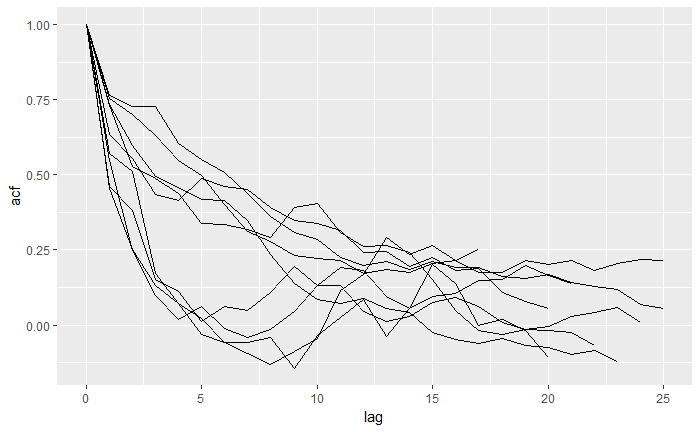## 3. Smoothing

The goal of smoothing is generally to aid visual interpretation of a time series. Let’s try on two subsets of our data.

``````test1 <- filter(poddata_day, Deployment_fk == 2578)
test2 <- filter(poddata_day, Deployment_fk == 2585)``````

Applying geom_smooth() plots a loess smoother (weighted regression) on the series.

``````ggplot(data = test1, aes(x = Time, y = Dpm)) +
geom_point() + geom_line() + geom_smooth() + ggtitle(2578)``````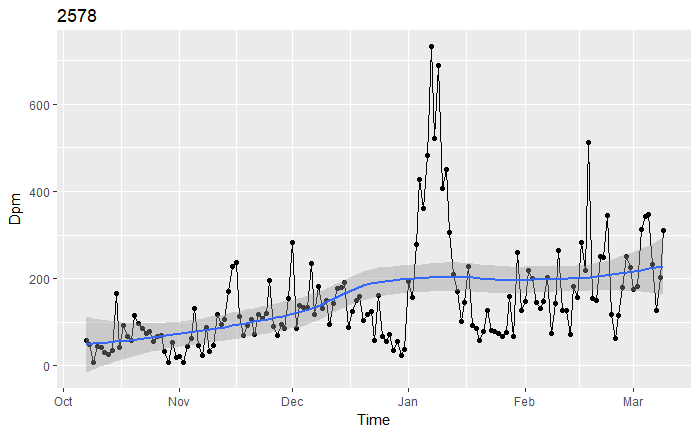``````ggplot(data = test2, aes(x = Time, y = Dpm)) +
geom_point() + geom_line() + geom_smooth() + ggtitle(2585)``````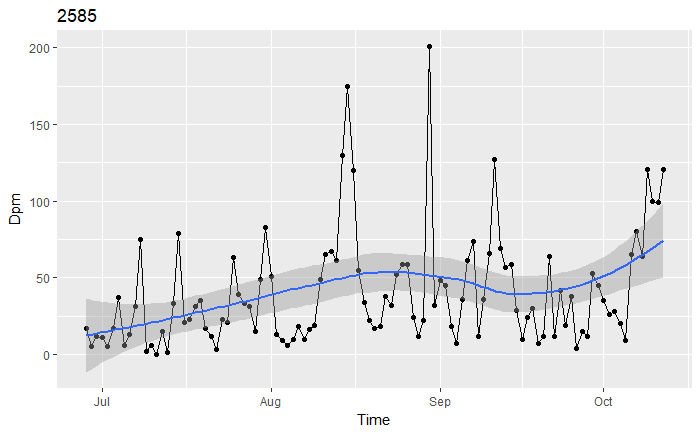Another way to smooth data, is to calculate a moving average.

``acf(test1\$Dpm) # First, we check the autocorrelation to choose our window size.``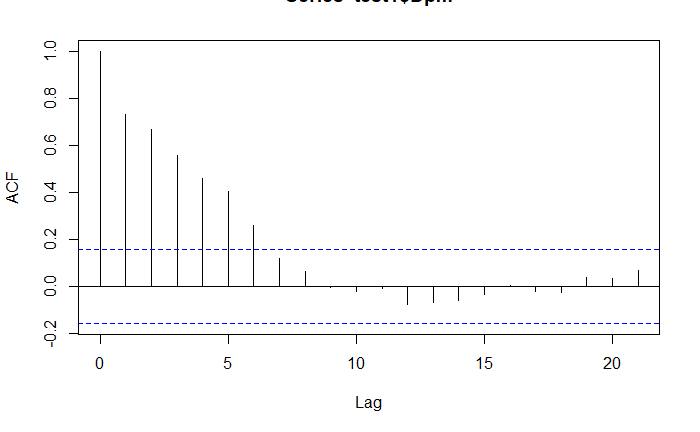``````library(pastecs)
``````
``````Loading required package: boot

Attaching package: <U+393C><U+3E31>pastecs<U+393C><U+3E32>

The following objects are masked from <U+393C><U+3E31>package:dplyr<U+393C><U+3E32>:

first, last``````
``````movavg <- decaverage(test1\$Dpm, order = 3) # By choosing an order of 3, a window size of 7 will be obtained (3 values to the left, 3 to the right).
movavg # movavg is a list``````
`````` Call:
decaverage(x = test1\$Dpm, order = 3)

Components
 "filtered"  "residuals"``````
``plot(movavg)``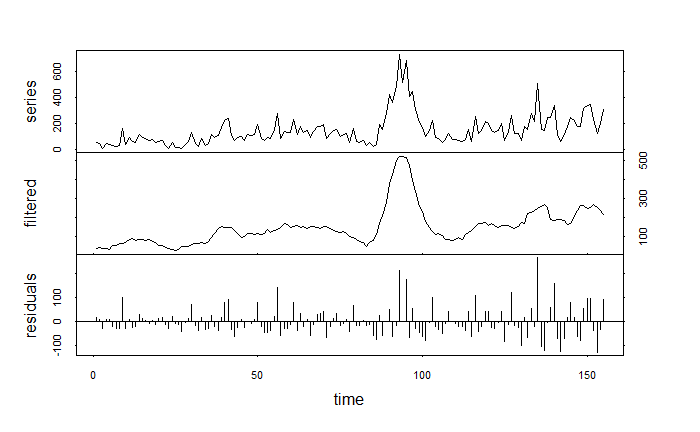Now in ggplot:

``test1\$decavg <- data.frame(movavg\$series)\$filtered``
``````ggplot(test1, aes(x = Time, y = Dpm)) + geom_point() + geom_line() +
geom_path(aes(y = decavg), size= 1.2, colour = "red")``````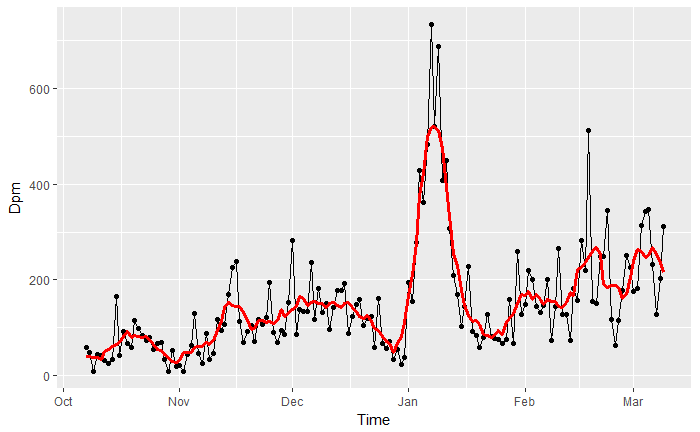``````movavg <- decaverage(test1\$Dpm, order = 3, times = 5)
test1\$decavg <- data.frame(movavg\$series)\$filtered``````
``````ggplot(test1, aes(x = Time, y = Dpm)) + geom_point() + geom_line() +
geom_path(aes(y = decavg), size= 1.2, colour = "red")``````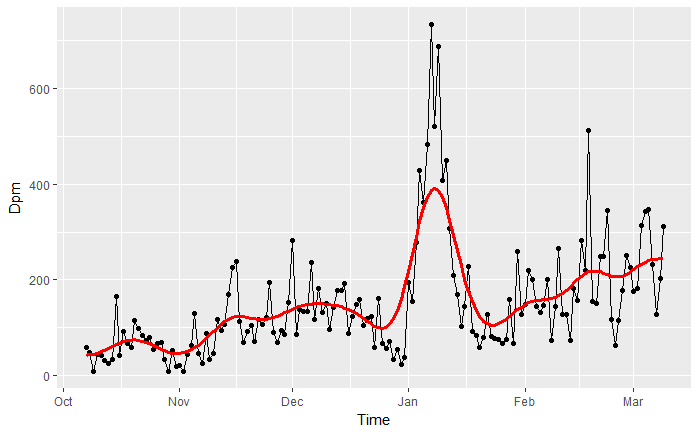Now, we can do the same for the other subset.

``acf(test2\$Dpm)``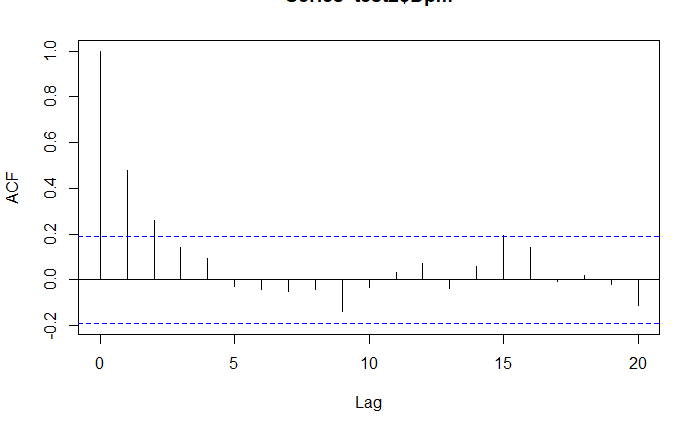``````movavg <- decaverage(test2\$Dpm, order = 1, times = 5)
test2\$decavg <- data.frame(movavg\$series)\$filtered``````
``````ggplot(test2, aes(x = Time, y = Dpm)) + geom_point() + geom_line() +
geom_path(aes(y = decavg), size= 1.2, colour = "red")``````